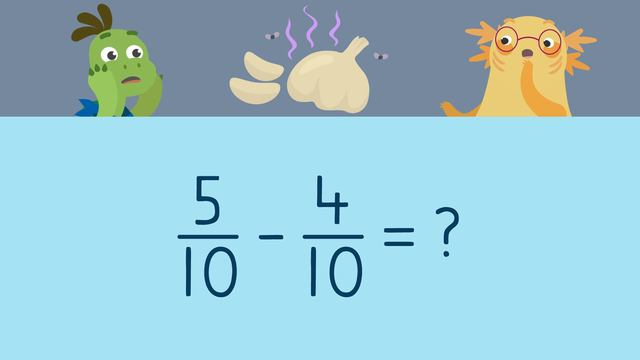# Subtracting Fractions with Like DenominatorsRate this video

Be the first to rate this video!

The authorTeam Digital
Subtracting Fractions with Like Denominators
CCSS.MATH.CONTENT.4.NF.B.3.A

## Information about the videoSubtracting Fractions with Like Denominators

### Contents

Axel and Tank are using a magic recipe to make an invisible spray. There's just one problem: They have to practice subtracting fractions in order to identify how much they need for each ingredient! We need to learn how to solve subtracting fractions in order to help Axel and Tank.

### Subtracting Fractions with Like Denominators

What is subtracting fractions with common denominators? Subtracting fractions with same denominator is similar to subtraction with whole numbers, but instead we are subtracting simple fractions. When subtracting fractions with like or common denominators, there are some steps for subtracting fractions that need to be followed. What are the rules for subtracting fractions? In fact, the rules for adding and subtracting fractions are very similar, the only difference is the operation which in this case is subtraction.

Subtracting fractions steps:

• Step 1: Check that the denominators are the same, and write it in the total
• Step 2: Subtract the numerators
• Step 3: Write the numerator above the denominator in the total

These steps are the rules for subtracting fractions with common denominators. Many have asked “when subtracting fractions do you subtract the denominator?” and the answer is no. The number of parts your whole is broken into does not change, so we never subtract the denominators when subtracting fractions with common denominators.

#### Subtracting Fractions Example

In the the video, you will see examples of subtracting fractions with like denominators. Let’s take a look at an example of subtracting fractions with same denominator. Here, we’ll subtract five-eighths minus two-eighths following the steps and rules for subtracting fractions with like denominators.

• Step 1: Check that the denominators are the same, and write it in the total

In this example, the denominators are eight, so the answer will have an eight as the denominator as well.• Step 2: Subtract the numerators

Here, the numerators are five and two, so we subtract them.

• Step 3: Write the numerator above the denominator in the total

Five minus two is three, so the answer is three-eighths.### Subtracting Fractions with Like Denominators Summary

If you forget what to do when subtracting fractions, always remember the steps for subtracting fractions that need to be followed. What are the rules for subtracting fractions? Below are the rules and steps to keep in mind when subtracting fractions with the same denominator.

Subtracting fractions steps:

• Step 1: Check that the denominators are the same, and write it in the total
• Step 2: Subtract the numerators
• Step 3: Write the numerator above the denominator in the total

Want some more subtracting fractions practice? In this video you can find subtracting fractions examples and on this website there are subtracting fractions with like denominators worksheets, along with other activities, and exercises.

### TranscriptSubtracting Fractions with Like Denominators

Axel and Tank are using a magic recipe to make an invisible spray. "We're going to be mixing invisible ink, Joyful Unicorn Tears, and garlic." "Yeah, but there's just one problem..." "we have to subtract fractions in order to identify how much we need of each ingredient!" Let's help Axel and Tank by, "Subtracting Fractions with Like Denominators", so they can make invisible spray! A fraction describes a part of a whole OR (...) a part of a collection. The top number, or the NUMERATOR, represents how many equal parts of a whole we have. The bottom number, or the DENOMINATOR, represents how many equal parts our whole is divided into. (...) A COMMON or LIKE denominator refers to fractions (...) with the SAME denominators. To start, we will subtract five-eighths and two-eighths to see how much invisible ink they need. When you subtract fractions with LIKE denominators... the denominator ALWAYS stays the same since the number of parts in our whole does NOT change. That is why our first step is to double check that the denominators are the SAME. Since they are LIKE denominators we can write the denominator of our total HERE. The second step is to subtract the numerators. Five minus two is three, and we write it HERE. (...) Axel and Tank need three-eighths of a container of invisible ink. Next, they will use Joyful Unicorn Tears. To determine the amount of tears, we will solve nine-twelfths minus three-twelfths. What is the first step? (...) First, double check that the denominators are the same... and write it HERE. What is the second step? (...) The second step is to subtract the numerators. What is nine minus three? (...) Six, so the answer is six-twelfths... which can also be simplified to one half. Axel and Tank need one-half of a container of Joyful Unicorn Tears. Last, we will subtract five-tenths minus four-tenths to see how much garlic they need. This time, try subtracting on your own. Pause the video so you have time to work (...) and press play again when you're ready to see the steps for finding the answer! First, double check that the denominators are the same... and write ten HERE. Next, subtract five and four to get... one. Axel and Tank will use one-tenth of a garlic bulb to complete their recipe! Before we see if the invisible spray works, let's summarize. Remember, (...) when subtracting fractions with LIKE denominators... The first step is to "double check that the denominators are the same, and write it in the total". The second step is to "subtract the numerators". The third step is to write the numerator above the denominator, simplifying the fraction when you need to. Let's see if the invisible spray works! "Axel, I can't see you anymore (...) THIS IS AWESOME!" "Yeah! Wait (...) do you smell (...) garlic!?" "Ew gross!"